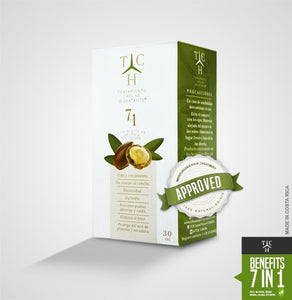# Tch Moisturizing Hair Treatment

• \$60.00
• Save \$15.05

• T

• C

• H

• g

• i

• v

• e

• s

• l

• i

• f

• e

• a

• n

• d

• g

• r

• o

• w

• t

• h

• ,

• g

• i

• v

• s

• b

• o

• d

• y

• t

• o

• h

• a

• i

• r

• ,

• e

• l

• a

• s

• t

• i

• c

• i

• t

• y

• ,

• s

• h

• i

• n

• e

• ,

• p

• r

• e

• v

• e

• n

• t

• s

• s

• p

• l

• i

• t

• e

• n

• d

• s

• a

• n

• d

• f

• a

• l

• l

• .

• p

• r

• o

• t

• e

• c

• t

• s

• f

• r

• o

• m

• t

• h

• e

• u

• s

• e

• o

• f

• t

• h

• e

• i

• r

• o

• n

• p

• l

• a

• t

• e

• .

• Color : transparent

TCH gives shine,life, softness,prevents fall and accelerates growth,minimizes frisss and prevents spit ends. Gives naturally straightness. Making a more lasting hair iron effect.

every pages